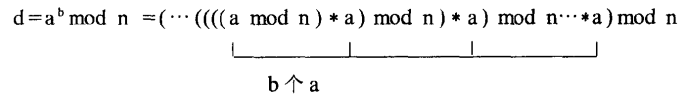//迭代法int modexp2(int a,int b,int n){int r;r
=a%n;for(int i=0;i<b-1;i++)r
=(r*a)%n;return r;}(a^b)%n=(a^（b/2）%n * a^（b/2）%n)%nint modExp(int a, int b, int n){int d=1,r=a;while(b){if(b%2==1){d=d*r%n;}r
=r*r%n;b
=b/2;}return d;}